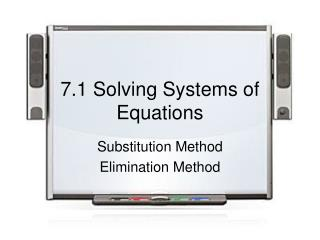DownloadDownload Presentation7.1 Solving Systems of Equations

7.1 Solving Systems of Equations

Download Presentation7.1 Solving Systems of Equations

- - - - - - - - - - - - - - - - - - - - - - - - - - - E N D - - - - - - - - - - - - - - - - - - - - - - - - - - -
Presentation Transcript

1. 7.1 Solving Systems of Equations Substitution Method Elimination Method

2. Substitution method Given as many equations as variables

3. Substitution method Given as many equations as variables

4. Substitution method Given as many equations as variables

5. Substitution method Given as many equations as variables

6. Elimination Method Given the Equations

7. Elimination Method Given the Equations

8. Elimination Method Given the Equations

9. Elimination Method Given the Equations

10. Elimination Method Given the Equations

11. Linear equations The intersection of two lines is one and only one point, unless the lines are parallel or the same line.

12. What is the Solution(s) if the lines are Parallel lines not meet, so no solution The Same line or lines that are onto, meet at all points on the lines, Infinitely many solutions.

13. Solve Find the Solutions

14. Solve Find the Solutions

15. Solve Find the Solutions

16. Solve Find all the values

17. Solve Find all the values

18. Solve Find all the values

19. Homework Page 483 # 4, 12, 20, 28, 36, 44, 52, 60, 72, 78, 86, 96

20. Homework Page 483 # 8, 16, 24, 32, 40, 48, 56, 68, 82, 90